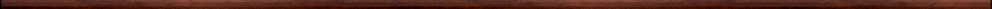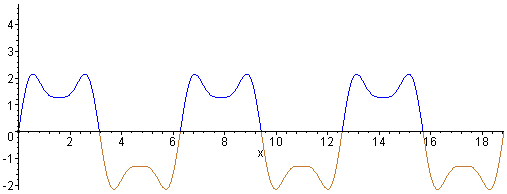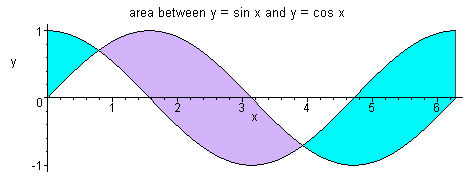Maple worksheets on integrationBasic calculus topics:

They are all compatible with Classic Worksheet Maple 10.Indefinite integrals - integ.mws

• The idea of integration.
• Rules for integration.
• Summary of standard integrals.
• Setting up a Maple procedure to perform basic integration.
• The Maple integration procedure: int.
• Examples.

Integration and area - defint.mws

• Antiderivatives, area and the Fundamental Theorem of Calculus.
• Examples of calculating areas under graphs.

The definite integral as a limit of a sum - defint2.mws

• Approximating areas under graphs by sums of areas of rectangles.
• Area elements and an explanation of the integral notation .. Riemann sums.
• Definite integrals of functions which may have negative values over the interval of integration

The definite integral as a limit of a sum - riemann.mws

• Summation formulas
• Integration using Riemann sums

A procedure for illustrating antiderivatives as area functions - areaplot.mws

• Graphs of antiderivatives as area functions.
• A procedure for plotting the area functions: areaplot.
• Examples

The area between two graphs - defint3.mws

• Calculating the area of a region between two graphs .. a special case.
• Examples of calculating the area of a region between two graphs.
• Calculating the area of a region between two graphs .. general case.
• More examples.

A procedure for illustrating the area between two graphs - areabtwn.mws

• A procedure for plotting the area enclosed between two graphs: areabetween.
• Sheering animation for area between graphs.
• Examples of calculating the area between two graphs.

Calculus procedures - calculus.zipTop of page

Main index